Math resources Geometry

3D shapes

Cone

# Cone

Here you will learn about cones, including how to classify and identify a cone, how to find the volume of a cone and how to find the surface area of a cone.

Students will first learn about a cone as part of geometry in 1 st grade. They will expand their learning in middle school and high school when they learn how to find the volume and surface area of a cone.

## What is a cone?

A cone is a three dimensional object that tapers from a circular base to a point. The term cone comes from the Greek word, “konos”, meaning a wedge or peak.

There is more than one type of cone, and the cone most commonly used is referred to as a “right circular cone”.

Examples of cones:

Right circular coneOblique cone

Real-life examples of cone like shapes include traffic cones, ice cream cones, volcano shapes, and party hats.

Parts of a cone:

Base

The base of the cone is a circle.

Vertex or apex

The vertex or apex of the cone is the point where all lateral sides meet.

Dimensions of a cone

The radius of the base of a cone is r .

The perpendicular height of a cone is h . The height of the cone is a line segment that connects the apex to the center of the circular base. It is perpendicular to the base of the cone.

The slant height of a cone is l . The slant height of the cone is the distance from any point on the base to the apex, along the curved surface of the cone.

## Volume of a cone

The volume of a cone is how much space there is inside a cone.

The formula for the volume of a cone is:

\text{Volume}=\cfrac{1}{3} \, \pi r^2 h

For example, find the volume of the cone, rounded to the nearest tenth.

\begin{aligned} \text { Volume of cone }&=\cfrac{1}{3} \, \pi r^2 h \\\\ & =\cfrac{1}{3} \times \pi \times 3^2 \times 4 \\\\ & =12 \pi \\\\ & =37.7 \mathrm{~cm}^3 \end{aligned}

## Surface area of a cone

The surface area of a cone is the area which covers the outer surface of a cone.

The surface area is made up of two parts, a curved surface area and a circular base.

The formula for calculating the curved surface area of a cone is:

\text{Curved surface area}=\pi rl

The formula for calculating the area of a circle:

\text{Area of circle}=\pi r^2

For the TOTAL surface area, you can add the two parts together:

\text{TOTAL surface area}=\pi rl+\pi r^2

For example, find the surface area rounded to the nearest tenth.

\text{Curved surface area}=\pi rl=\pi \times 3\times 5=15\pi

\text{Area of circle}=\pi r^2=\pi \times 3^2=9\pi

\text{TOTAL surface area}= 15\pi + 9\pi = 24\pi = 75.4 {~cm}^2

### What is a cone?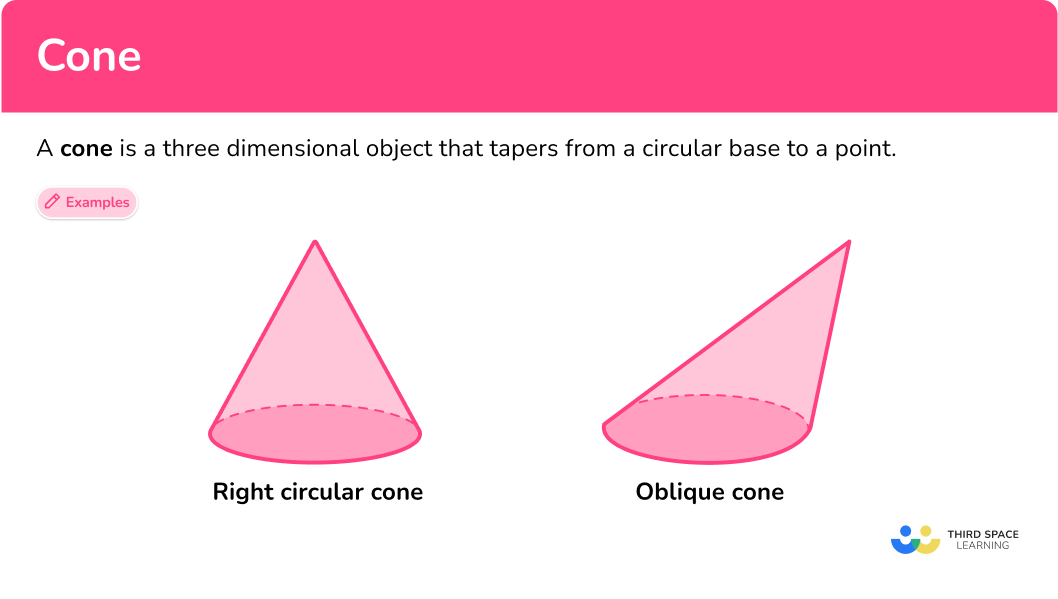## Common Core State Standards

How does this relate to 1 st, 7 th and 8 th grade math?

Compose two-dimensional shapes (rectangles, squares, trapezoids, triangles, half-circles, and quarter-circles) or three-dimensional shapes (cubes, right rectangular prisms, right circular cones, and right circular cylinders) to create a composite shape, and compose new shapes from the composite shape.

Solve real-world and mathematical problems involving area, volume and surface area of two- and three-dimensional objects composed of triangles, quadrilaterals, polygons, cubes, and right prisms.

Know the formulas for the volumes of cones, cylinders, and spheres and use them to solve real-world and mathematical problems.

## How to identify a cone

In order to identify a cone, you will:

1. Look for the characteristics of a cone.
2. State whether or not the shape is a cone.
3. If the shape is not a cone, explain what characteristics are different.

## Cone examples

### Example 1: cone example

Look at the image below and determine if it is a cone or not.

1. Look for the characteristics of a cone.

A cone has a circular base with a curved surface area that meets at a vertex that is directly above the center of the circular base.

This shape also has a circular base and a curved surface area that meets at a vertex.

2State whether or not the shape is a cone.

This shape is a cone because it has a circular base with a curved surface that meets at a vertex.

### Example 2: cone example

Look at the image below and determine if it is a cone or not.

A cone has a circular base with a curved surface area that meets at a vertex that is directly above the center of the circular base.

This shape has a rectangular base with 4 triangular faces that meet at a vertex.

This shape is a pyramid, not a cone.

This shape has a rectangular base with 4 triangular faces that meet at a vertex.

## How to calculate the volume of a cone

In order to calculate the volume of a cone:

1. Write down the formula.
2. Substitute the given values.
3. Calculate the volume of the cone.
4. Write the final answer, including the units.

## Volume of a cone examples

### Example 3: volume of a cone

Find the volume of the cone with radius 5.3{~cm} and perpendicular height 7.8{~cm} .

\text {Volume }=\cfrac{1}{3} \, \pi r^{2} h

\begin{aligned} & r=5.3 \\\\ & h=7.8 \end{aligned}

\begin{aligned} \text { Volume } &=\cfrac{1}{3} \, \pi r^{2} h \\\\ &=\cfrac{1}{3} \times \pi \times 5.3^{2} \times 7.8 \end{aligned}

\begin{aligned} &=\cfrac{1}{3} \times \, \pi \times 5.3^{2} \times 7.8 \\\\ &=229.443 \ldots \end{aligned}

The answer rounded to the nearest centimeter is V = 229 {~cm}^3 .

### Example 4: volume of a cone

Find the volume of the cone with radius 9{~cm} and perpendicular height 11{~cm} .

\text {Volume }=\cfrac{1}{3} \, \pi r^{2} h

\begin{aligned} & r=9 \\\\ & h=11 \end{aligned}

\begin{aligned} \text { Volume } &=\cfrac{1}{3} \, \pi r^{2} h \\\\ &=\cfrac{1}{3} \times \pi \times 9^{2} \times 11 \end{aligned}

\begin{aligned} &=\cfrac{1}{3} \times \, \pi \times 9^{2} \times 11 \\\\ &=297 \pi \end{aligned}

=297 \pi {~cm}^3

## How to calculate the surface area of a cone

In order to calculate the surface area of a cone:

1. Calculate the area of each face.
2. Add the area of each face together.
3. Include the units.

## Surface area of a cone examples

### Example 5: total surface area of a cone

Find the curved surface area of the cone with radius 4.3{~cm} and slant height 9.6{~cm} .

\begin{aligned} \text {Curved surface area} &= \, \pi r l \\\\ &=\pi \times 4.3 \times 9.6 \\\\ &=129.6849 \ldots \\\\ &=129.7 \text { (round to the nearest tenth) } \end{aligned}

\begin{aligned} \text {Area of circle } &= \, \pi r^2 \\\\ &=\pi \times 4.3^2 \\\\ &=58.0880 \ldots \\\\ &=58.1 \text { (rounded to the nearest tenth) } \end{aligned}

Total surface area: 129.7+58.1=187.8

Surface area =188 \; cm^2

### Example 6: total surface area of a cone

Find the curved surface area (lateral area) of the cone with radius 8{~cm} and slant height 13{~cm} .

\begin{aligned} \text{Curved surface area}&=\, \pi rl\\\\ &=\pi \times 8 \times 13\\\\ &= 104 \pi \end{aligned}

\begin{aligned} \text{Area of circle }&=\, \pi r^2\\\\ &=\pi \times 8^2\\\\ &=64\pi \end{aligned}

Total surface area: 104\pi +64\pi = 168\pi

=168 \pi \mathrm{~cm}^{2}

### Teaching tips for cone

• Introduce and reinforce important vocabulary words, such as base, vertex, height, slant height, lateral surface area, and volume. Make sure to encourage students to use these terms correctly when discussing cones.

• Provide hands-on activities for students to explore and manipulate cone shapes. For example, you can give them playdough or clay to create their own cones, or ask them to cut out and fold paper cones.

• Allow students to practice calculating the volume and surface area of cones. You can also give them worksheets or problem-solving tasks involving cone calculations to reinforce their understanding.

### Easy mistakes to make

• Incorrectly applying a formula
There are several formulas that can be used, so you would need to match the correct formula to the correct context.
For example, it would be easy to mix up \pi r^2 + \pi r , the formula to find the area of a circle, and the formula to find the volume of a cone,
\text {Volume }=\cfrac{1}{3} \, \pi r^2 h.

• Confusing the radius and diameter
It is a common error to mix up radius and diameter. Remember, the radius of the cone is half of the distance across the circular base, and the diameter is the full distance across the circular base.
For example,

• Use of incorrect units
It’s easy for students to mix up when to use the different types of units. For area, you use square units such as {~cm}^2 , and for volume, you use cubic units such as {~cm}^3 .

### Practice cone questions

1) Which shape is an example of a cone?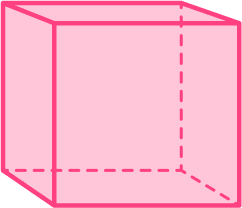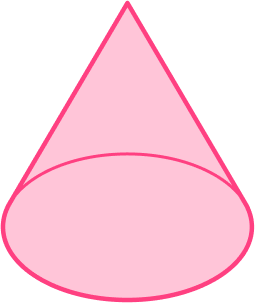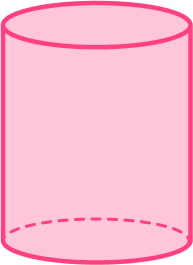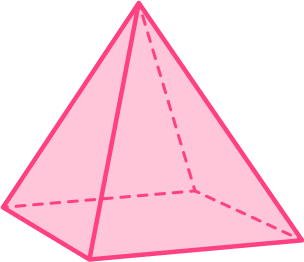This shape has a circular base with a curved surface area that meets at a vertex that is directly above the center of the circular base.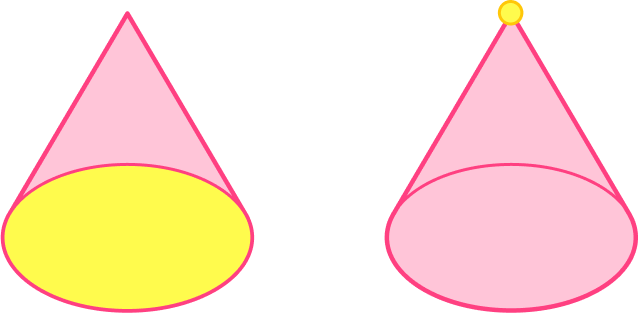This shape is a cone.

2) Which shape is an example of a cone?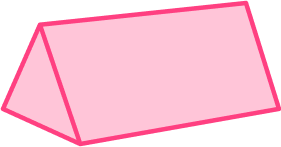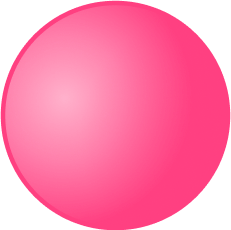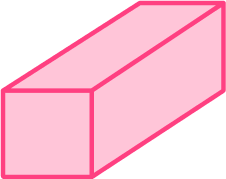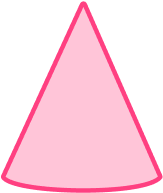This shape has a circular base with a curved surface area that meets at a vertex that is directly above the center of the circular base.This shape is a cone.

3) Find the volume of a cone to the nearest whole cubic centimeter with a radius of 9.4 {~cm} and perpendicular height of 8.7 {~cm} .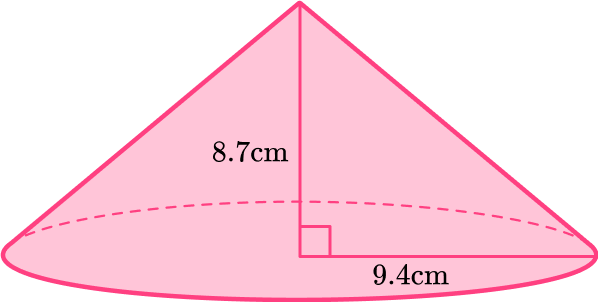805 {~cm}^3806 {~cm}^3745 {~cm}^3746 {~cm}^3Find the volume of a cone so you can substitute the values of r and h into the formula.

\begin{aligned} & r=9.4 \\\\ & h=8.7 \end{aligned}

\begin{aligned} & V=\cfrac{1}{3} \, \pi r^2 h \\\\ & V=\cfrac{1}{3} \times \pi \times 9.4^2 \times 8.7 \end{aligned}

V=805.014 \ldots

( 805.014 rounded to the nearest whole number would give you…)

V=805 \mathrm{~cm}^3

4) Find the volume of a cone of radius 8 {~cm} and perpendicular height 6 {~cm} . Leave your answer in terms of \pi .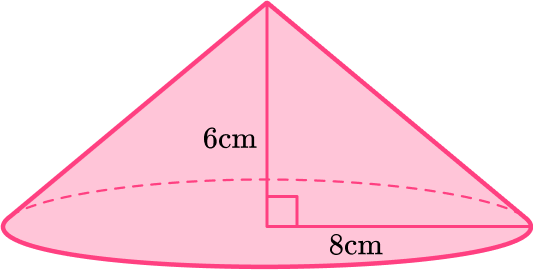127 \pi {~cm}^3126 \pi {~cm}^3128 \pi {~cm}^3125 \pi {~cm}^3Find the volume of a cone so you can substitute the values of r and h into the formula.

\begin{aligned} & r=8 \\\\ & h=6 \\\\ & V=\cfrac{1}{3} \, \pi r^2 h \\\\ & V=\cfrac{1}{3} \times \pi \times 8^2 \times 6 \\\\ & V=128 \pi \\\\ & V=128 \pi \mathrm{~cm}^3 \end{aligned}

5) Find the curved surface area (lateral area) of a cone of radius 4.3 {~cm} and slant height 6.2 {~cm} .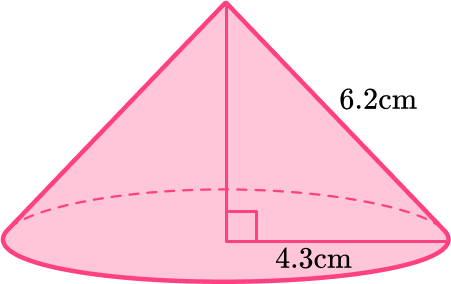83.7 {~cm}^2360.1 {~cm}^2360.2 {~cm}^283.8 {~cm}^2You will find the curved surface area of a cone, so you can substitute the values of r and h into the formula.

\begin{aligned} \text{Curved surface area}&= \, \pi r l \\\\ & =\pi \times 4.3 \times 6.2 \\\\ & =83.754 \ldots \text{ (round to the nearest tenth)}\\\\ & =83.8 \mathrm{~cm}^2 \end{aligned}

6) Find the curved surface area (lateral area) of a cone of radius 7 {~cm} and slant height 9 {~cm} . Leave your answer in terms of \pi .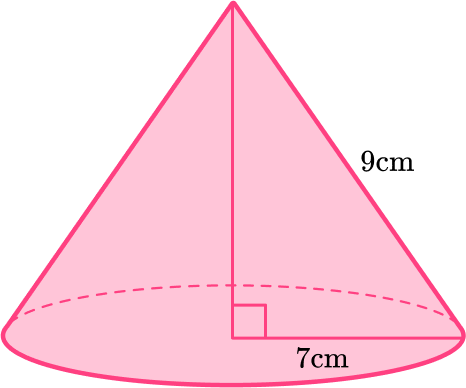61 \pi{~cm}^263 \pi{~cm}^262 \pi{~cm}^264 \pi{~cm}^2You are finding the curved surface area of a cone, so substitute the values of r and l into the formula.

\begin{aligned} \text{Curved surface area}&= \,\pi rl\\\\ &=\pi \times 7\times 9\\\\ &=63\pi\\\\ &=63\pi \ cm^2\\\\ \end{aligned}

## Cone FAQs

What is the difference between a right circular cone and an oblique cone?

A right circular cone is the most common type of cone. It has a circular base, and the apex is directly above the center of the base. The axis of the cone is perpendicular to the base, creating a right angle. An oblique cone is any cone that is not a right circular cone. In an oblique cone, the axis is not perpendicular to the base, resulting in a slanted shape.

What are the different types of cones?

During your math journey, you will encounter right circular cones, oblique cones, acute cones, and obtuse cones.

## Still stuck?

At Third Space Learning, we specialize in helping teachers and school leaders to provide personalized math support for more of their students through high-quality, online one-on-one math tutoring delivered by subject experts.

Each week, our tutors support thousands of students who are at risk of not meeting their grade-level expectations, and help accelerate their progress and boost their confidence.